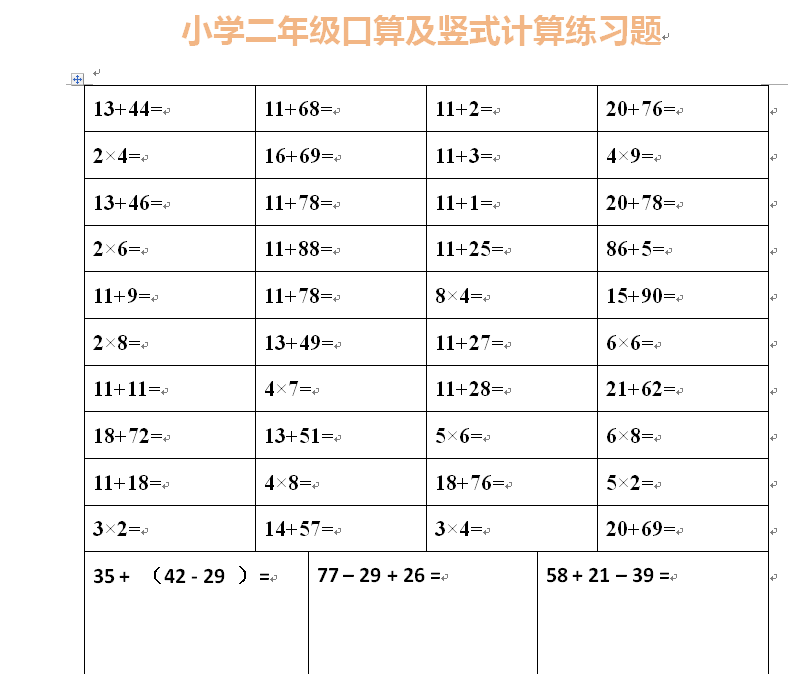13+44= 11+68= 11+2= 20+76= 2×4= 16+69= 11+3= 4×9= 13+46= 11+78= 11+1= 20+78= 2×6= 11+88= 11+25= 86+5= 11+9= 11+78= 8×4= 15+90=##### 评论信息